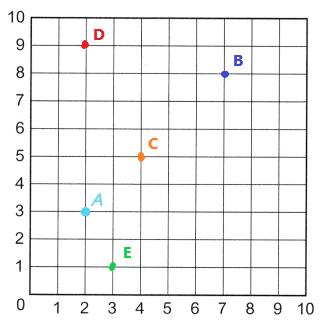# McGraw Hill Math Grade 5 Chapter 11 Lesson 3 Answer Key Plotting Points on a Coordinate Grid

All the solutions provided in McGraw Hill Math Grade 5 Answer Key PDF Chapter 11 Lesson 3 Plotting Points on a Coordinate Grid are as per the latest syllabus guidelines.

## McGraw-Hill Math Grade 5 Answer Key Chapter 11 Lesson 3 Plotting Points on a Coordinate Grid

Plot and Label

Plot and label each point on the grid. The first point has been plotted and labeled for you.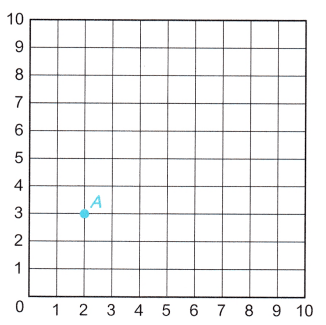Question 1.
A (2, 3)The point A (2, 3) is labeled and plotted on the grid.

Question 2.
B (7, 8)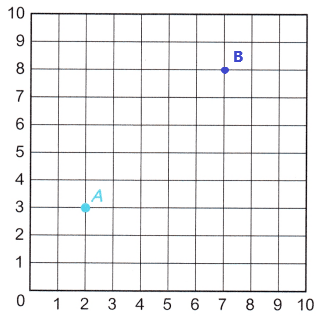The point B (7, 8) is labeled and plotted on the grid.

Question 3.
C (4, 5)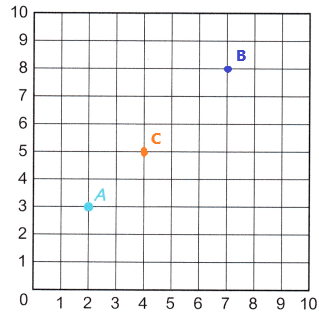The point C (4, 5) is labeled and plotted on the grid.

Question 4.
D (2, 9)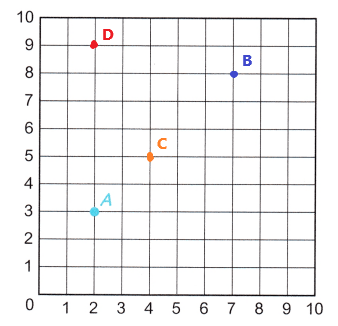The point D (2, 9) is labeled and plotted on the grid.

Question 5.
E (3, 1)